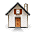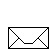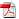Faqs | Help | @ | Contact |## 2-envelope puzzle

For the time being ....During the summer break of 2006/7, I spent some time working on the famous Two-Envelope Paradox. I embarked on this project after reading the WIKIPEDIA entry on this topic.

I was greatly dissatisfied with the discussion that was posted there at the time (4:37AM, January 20, 2007 ).

So, I buckled down and formulated my thoughts on this topic in a paper that may be of interest to you:The Two-Envelopes Paradox: A Primer for DummiesThe paper contains some interesting results.Here is a description of the puzzle — taken from WIKIPEDIA:

The player is given two indistinguishable envelopes, each of which contains a positive sum of money. One envelope contains twice as much as the other. The player may select one envelope and keep whatever amount it contains, but upon selection, is offered the possibility to take the other envelope instead.

The switching argument:

1. Denote by A the amount in the selected envelope.
2. The probability that A is the smaller amount is 1/2, and that it's the larger also 1/2.
3. The other envelope may contain either 2A or A/2.
4. If A is the smaller amount, the other envelope contains 2A.
5. If A is the larger amount, the other envelope contains A/2.
6. Thus, the other envelope contains 2A with probability 1/2 and A/2 with probability 1/2.
7. So the expected value of the money in the other envelope is: (1/2)2A + (1/2)(A/2) = (5/4)A.
8. This is greater than A, so swapping is favored.
9. After the switch, reason in exactly the same manner as above, but denote the second envelope's contents as B.
10. It follows that the most rational thing to do is to swap back again.
11. This line of reasoning dictates that envelopes be swapped indefinitely.
12. As it seems more rational to open just any envelope than to swap indefinitely, the player is left with a paradox.The puzzle:
The puzzle is to find the flaw, the erroneous step, in the switching argument above. This includes determining exactly why and under what conditions that step is not correct, in order to be sure not to make this mistake in a more complicated situation where the misstep may not be so obvious. In short, the problem is to solve the paradox.

Remark (mine):
Observe that the objective is not to show that ... there is no advantage in swapping envelopes. We know, and it is easy to explain why, this is so. Rather, the objective is to identify the error in the logical progression outlined above. To find the exact point, in this progression, at which the error is made.

It is interesting that the debate about the "correct" solution to this puzzle is ongoing and is as lively as ever.

Disclaimer: This page, its contents and style, are the responsibility of the author (Moshe Sniedovich) and do not represent the views, policies or opinions of the organizations he is associated/affiliated with.